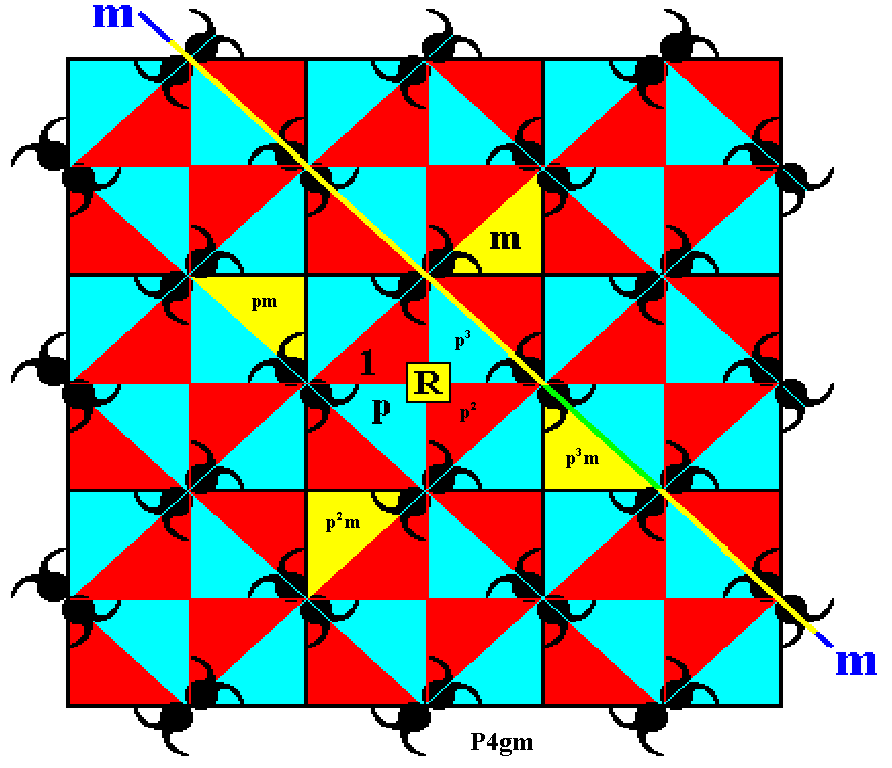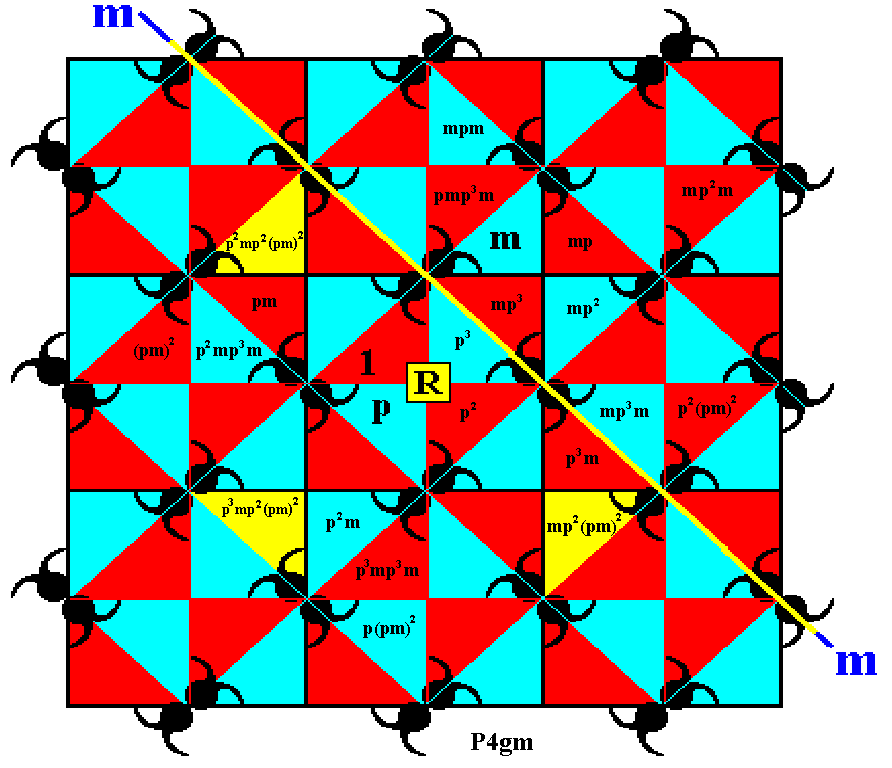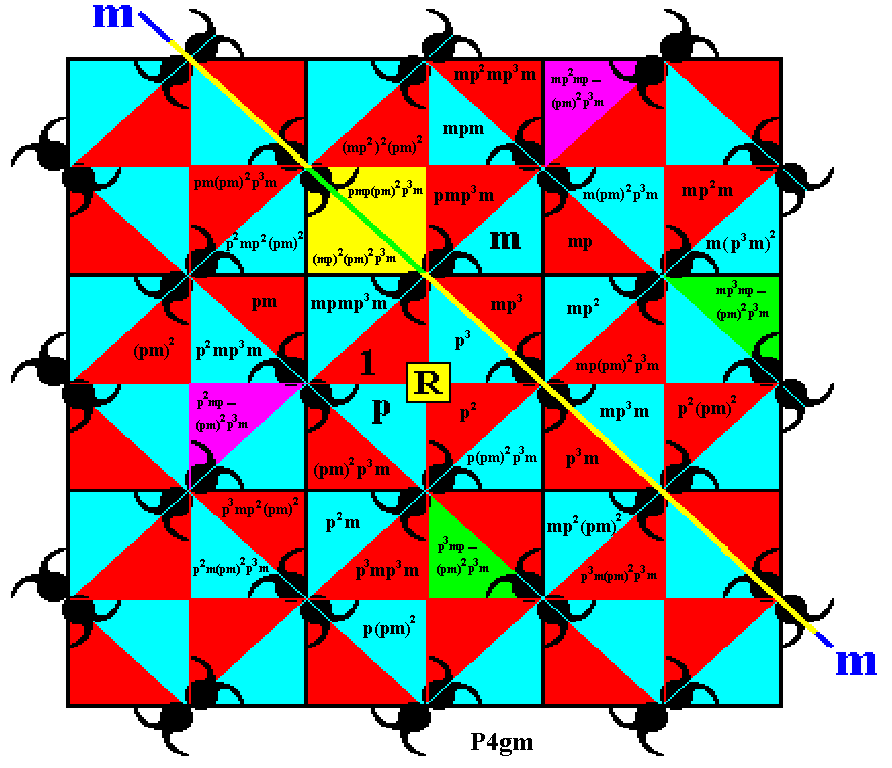```

```
Wholeness and the Implicate Order
```
```
The Crystallization Process and the Implicate Order
(Part Nine)

```

```
We  will continue our investigation concerning the generation of the Plane Group P4gm, i.e. the generation of its group elements.
```
```
In the partitioned P4gm pattern of the previous document we now explicitly indicate an initial group element and two generators.Figure 1.  The pattern representing Plane Group  P4gm  is partitioned (as it had been already in the previous document) into triangular areas representing group elements. Three such group elements are explicitly indicated and also the relevant symmetry elements (rotation axis and mirror line) associated with the generators.

```
```
In the following -- where we generate the group elements -- we shall use an enlarged version of the above Figure in order to have enough space for the markings of group elements.

First we generate the elements  p2  and  p3  by repeatedly applying the generator  p  -- which is an anticlockwise rotation of 900 about the point  R  -- to the initial group element (motif unit s.l.).Figure 2.  Generation of the group elements  p2  and  p3 .

```
```Figure 3.  Rotating the element  m  anticlockwise by resp. 900, 1800 and 2700 we obtain the elements  pm ,  p2m  and  p3m .

```
```Figure 4.  New group elements are generated by applying the mirror reflection  m  to already existing elements.

```
```Figure 5.  More group elements are generated by (repeatedly) applying  p  (anticlockwise rotation of 900 about the point  R ) to already existing elements.

```
```Figure 6.  Still more group elements are generated by (repeatedly) applying  p  (anticlockwise rotation of 900 about the point  R ) to already existing elements.

```
```Figure 7.  One new element is generated by applying the reflection  m

```
```Figure 8.  Two more group elements are generated by (repeatedly) applying  p  (anticlockwise rotation of 900 about the point  R ) to already existing elements.

```
```Figure 9.  Some new elements are generated by applying the reflection  m  to already existing elements.

```
```Figure 10.  Two more group elements are generated by (repeatedly) applying  p  (anticlockwise rotation of 900 about the point  R ) to an already existing element.

```
```Figure 11.  Some new elements are generated by applying the reflection  m  to already existing elements.

```
```Figure 12.  Three more group elements are generated by (repeatedly) applying  p  (anticlockwise rotation of 900 about the point  R ) to an already existing element.

```
```Figure 13.  Again, three more group elements are generated by (repeatedly) applying  p  to an already existing element.

```
```Figure 14.  Three new elements are generated by applying the reflection  m  to already existing elements.

```
```Figure 15.  Again, two more group elements are generated by (repeatedly) applying  p  to an already existing element.

```

```
In the next document we will continue (and conclude) the generation of the group elements of our group P4gm.

e-mail :```
```
To continue click HERE for further study of the totally dynamic and holistic nature of Reality.
```
```
back to homepage

****************